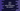# How to print a Hollow Rhombus pattern in Java## How to print a Hollow Rhombus pattern in Java:

In this post, we will learn how to print a hollow Rhombus pattern with star in Java. I will show you how the algorithm works and how to write it in Java.

### Algorithm to print a Rhombus:

A Rhombus of height 10 drawn with stars looks as below:

``````         * * * * * * * * * *
*                 *
*                 *
*                 *
*                 *
*                 *
*                 *
*                 *
*                 *
* * * * * * * * * * ``````

To understand how it works, let’s try it with a small Rhombus:

``````    * * * * *
*       *
*       *
*       *
* * * * * ``````

This is a Rhombus of height 5. We are using stars and blank spaces to print this pattern. Let me replace all blank spaces with #:

``````####* * * * *
###* # # # *
##* # # # *
#* # # # *
* * * * * ``````

If you look closely,

• On each row, it prints #, stars or # and stars.
• We can run one for loop from i = 1 to i = height
• Inside this loop, run two for loops, the first one will print the starting blank spaces and the second one will print stars and blank spaces.
• The first inner loop will run from j = 1 to j = height - i. On each iteration, it will print a blank space.
• The second inner loop will run from k = 1 to k = height. If the value of i or k is 1 or height, it will print a star, else it will print a blank space on each iteration.

### Example 1: Java program to print a hollow Rhombus with for loops:

The below Java program prints a hollow Rhombus pattern with user-input height value:

``````import java.util.Scanner;

public class Main {

public static void main(String[] args) {
Scanner scanner = new Scanner(System.in);
System.out.println("Enter the height of the Rhombus: ");

int height = scanner.nextInt();

for (int i = 1; i <= height; i++) {
for (int j = 1; j <= height - i; j++) {
System.out.print(" ");
}
for (int k = 1; k <= height; k++) {
if (i == 1 || i == height || k == 1 || k == height) {
System.out.print("* ");
} else {
System.out.print("  ");
}
}
System.out.println();
}
}
}``````

It reads the height of the Rhombus and assigns it to the variable height. It is following the same algorithm we discussed above. You can run this program to print a Rhombus of any height.

``````Enter the height of the Rhombus:
10
* * * * * * * * * *
*                 *
*                 *
*                 *
*                 *
*                 *
*                 *
*                 *
*                 *
* * * * * * * * * * ``````

### Example 2: Java program to print a hollow Rhombus with while loops:

We can re-write the above program with while loops. We have to initialize the variable before the loop starts and increment the value at the end of each iteration in the loop.

``````import java.util.Scanner;

public class Main {

public static void main(String[] args) {
Scanner scanner = new Scanner(System.in);
System.out.println("Enter the height of the Rhombus: ");

int height = scanner.nextInt();
int i = 1, j, k;

while (i <= height) {
j = 1;
while (j <= height - i) {
System.out.print(" ");
j++;
}

k = 1;
while (k <= height) {
if (i == 1 || i == height || k == 1 || k == height) {
System.out.print("* ");
} else {
System.out.print("  ");
}
k++;
}
System.out.println();
i++;
}
}
}``````

This works exactly like the above program. You will get similar result.

``````Enter the height of the Rhombus:
6
* * * * * *
*         *
*         *
*         *
*         *
* * * * * * ``````